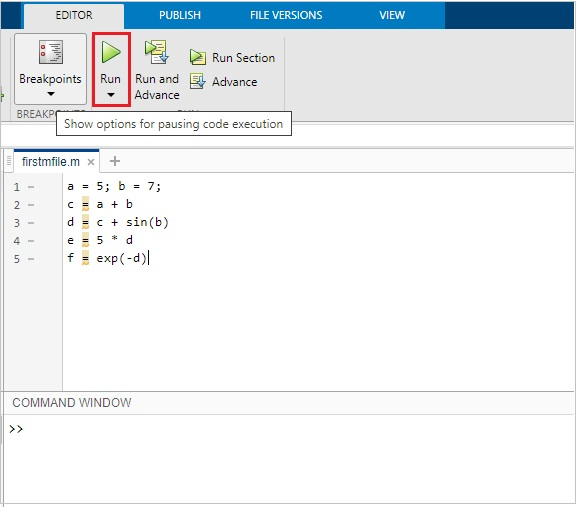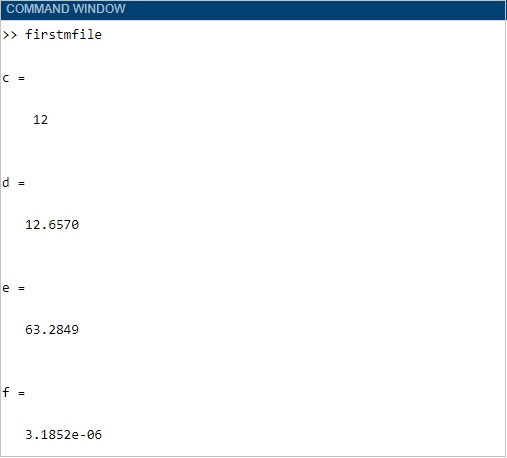# Matlab M-Files - Run

In the previous chapter, we have seen how to create and save a m-file. Now in this chapter, we will write a simple code and run the same.

Following is the code which will run inside the firstmfile.m

```a = 5; b = 7;
c = a + b
d = c + sin(b)
e = 5 * d
f = exp(-d)
```

This is how it will look inside MATLAB editor −Click on the Run button as highlighted above to see the result inside the command window as shown below